# Disco Inferno

Oh yeah, this was fun. At first, coming up with *any* one concept for a looping animation felt like a daunting task, especially after witnessing an overwhelming breadth of possibilities by Bees & Bombs and other genius finessers on the internet. Soon however, I found myself honing in on concepts that would illustrate the sensation of infinity that you feel when watching looping animations. My aesthetic preferences are commonly derived from geometry in science and nature so the first form that I thought resonated best with this theme was the Mobius Strip. It was also a form that I was familiar representing in the past, but never specifically in a text-based programming environment.

What was interesting about making this form selection was that I could have never anticipated that this is what it would turn out looking like. It was extremely exciting to 1. understand the parametric construction of the illusory Mobius in Processing 2. Use my understanding of it’s parametric construction to build out engaging languages of motion and color 3. Use a tool that allowed me to rapidly iterate and finesse aesthetic details in my composition, unlike basically any video software in Adobe CC.

What I really like about the outcome was the effect that the easing had on the Mobius construction, which continuously reveals and conjoins the individual spheres that form the vertical lines. I also appreciate that the general movement and color is reminiscent of something out of a disco. That being said, there are many things to be improved here, such as the start and end positions of the Mobius in this recording of the gif, as well as changing the camera angle to be a bit more engaged with the form.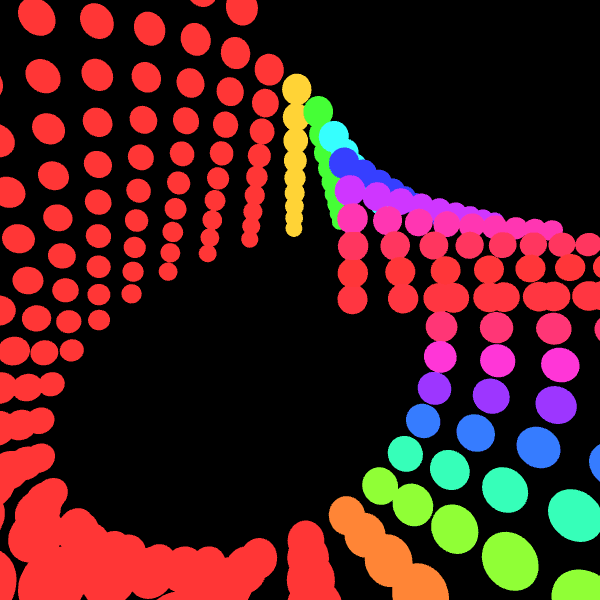Thank you to Golan for getting me started on the Mobius construction, and to Aman for helping me with some of the vertex and color math! Mobius algorithm from Paul Bourke.

## Process Documentation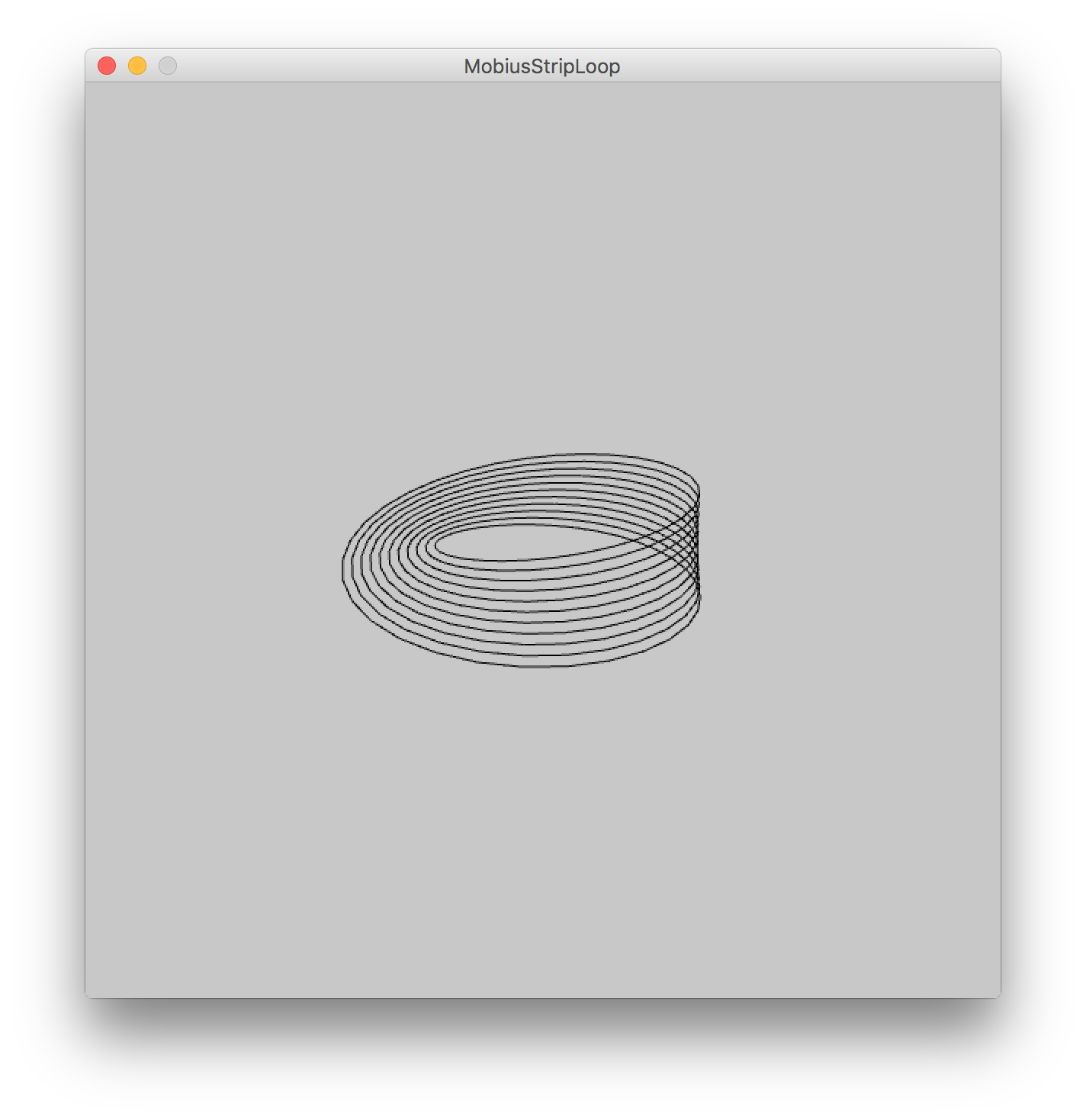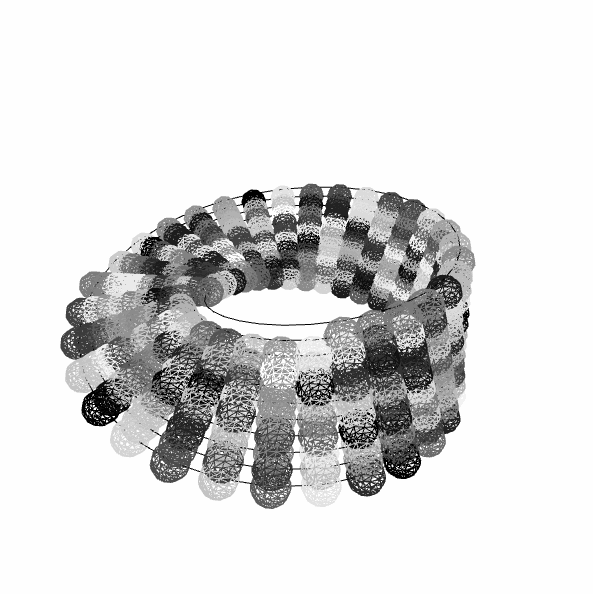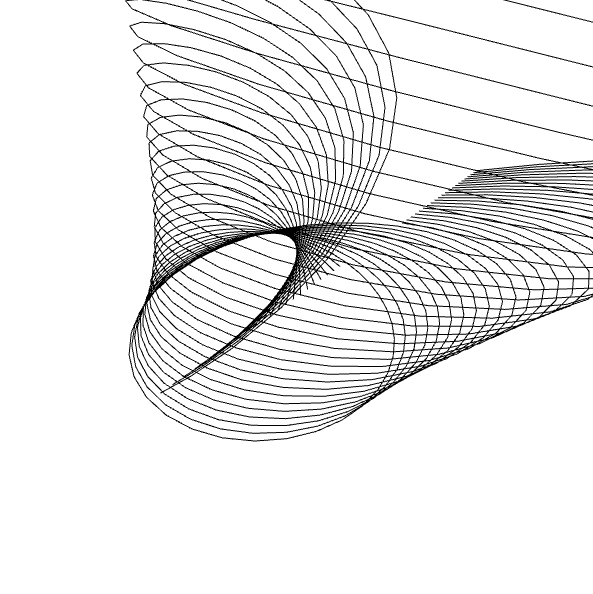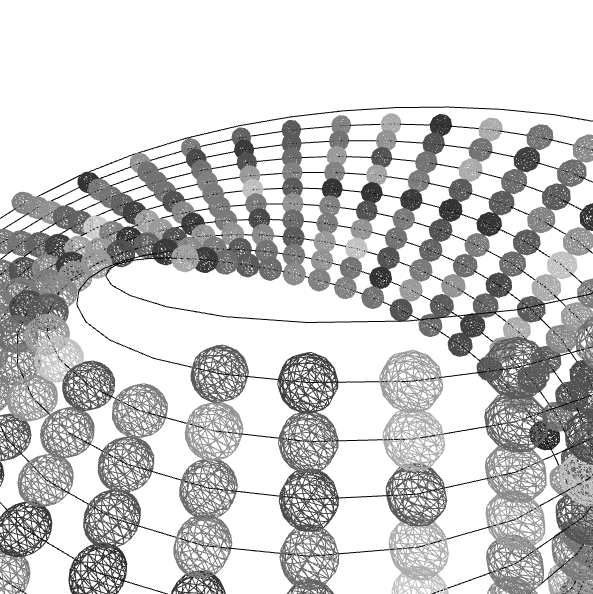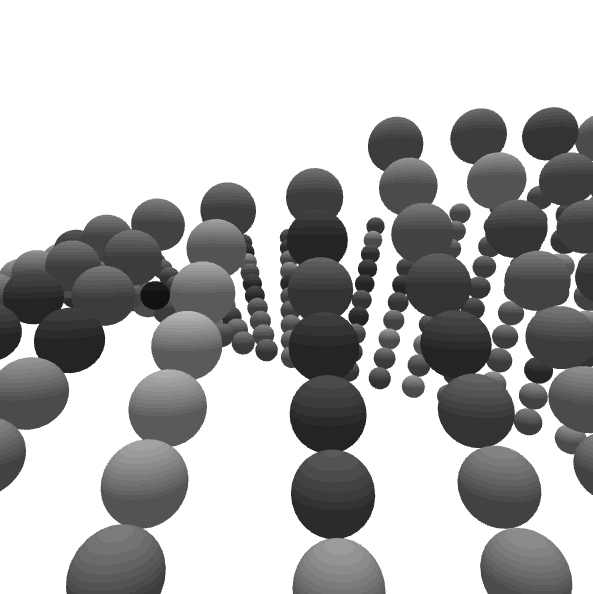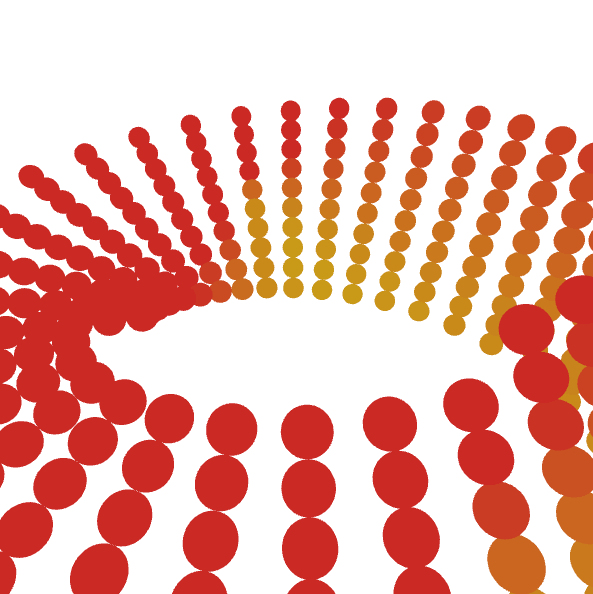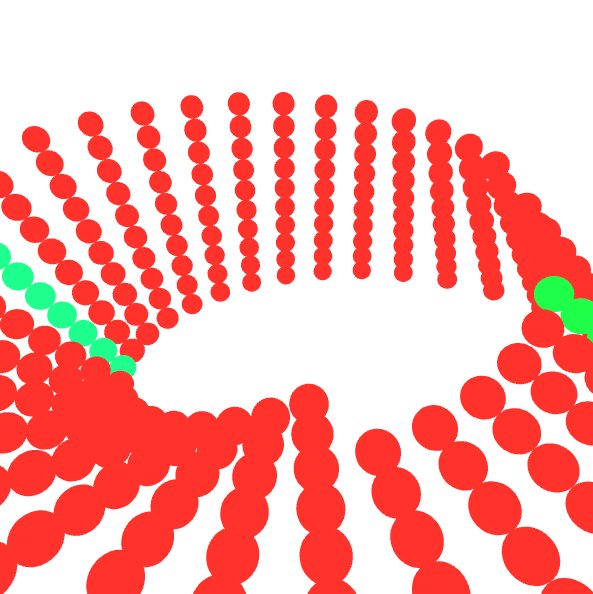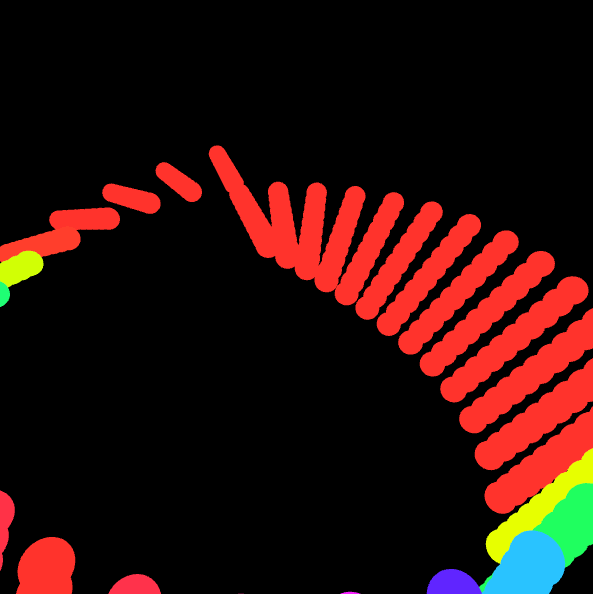```// This is a template for creating a looping animation in Processing/Java. // When you press the 'F' key, this program will export a series of images // into a "frames" directory located in its sketch folder. // These can then be combined into an animated gif. // Known to work with Processing 3.3.6 // Prof. Golan Levin, January 2018   float t; float [] xa, ya, za; //float frameCount;   //=================================================== // Global variables. String myNickname = "agusman"; int nFramesInLoop = 240; int nElapsedFrames; boolean bRecording;   //=================================================== void setup() { // size (500, 200); bRecording = false; nElapsedFrames = 0; size(600, 600, P3D); frameRate(30); lights(); ambientLight(255, 255, 255); colorMode(HSB, 255); } //=================================================== void keyPressed() { if ((key == 'f') || (key == 'F')) { bRecording = true; nElapsedFrames = 0; } }   //=================================================== void draw() {   // Compute a percentage (0...1) representing where we are in the loop. float percentCompleteFraction = 0; if (bRecording) { percentCompleteFraction = (float) nElapsedFrames / (float)nFramesInLoop; } else { percentCompleteFraction = (float) (frameCount % nFramesInLoop) / (float)nFramesInLoop; }   // Render the design, based on that percentage. renderMyDesign (percentCompleteFraction);   // If we're recording the output, save the frame to a file. if (bRecording) { saveFrame("MobiusBoy/" + myNickname + "_frame_" + nf(nElapsedFrames, 4) + ".png"); nElapsedFrames++; if (nElapsedFrames >= nFramesInLoop) { bRecording = false; } } }   //=================================================== void renderMyDesign (float percent) { // // YOUR ART GOES HERE. // This is an example of a function that renders a temporally looping design. // It takes a "percent", between 0 and 1, indicating where we are in the loop. // This example uses two different graphical techniques. // Use or delete whatever you prefer from this example. // Remember to SKETCH FIRST! //https://www.youtube.com/watch?v=jftxZ64m42I   background(255); //background(cos(atan2(frameCount, frameCount) + (map(percent, 0, 1, 0, 1F* TWO_PI))) * 255, 200, 255); pushMatrix(); translate(width/2, height/2); rotateX(1); //rotateY(frameCount); rotateZ(map(percent, 0, 1, 0, TWO_PI));   int nPoints = 32; int nLayers = 10; float w = 150; float R = 300; noFill();   ////rotate mobius strip //frameCount = frameCount + 0.05;     //arrays to store some quanitity of points per perpendicular line on strip xa = new float [33*11]; ya = new float [33*11]; za = new float [33*11]; int index = 0;   //http://mathworld.wolfram.com/MoebiusStrip.html for (int j=0; j<=nLayers; j++) { float s = map(j, 0, nLayers, -w + sin(map(percent, 0, 1, 0, (2*TWO_PI))) * 400, w + cos(map(percent, 0, 1, 0, (3* TWO_PI))) * 100); noStroke(); //stroke(0); beginShape();   //run vertexes through 360 degrees of a circle for (int i=0; i<=nPoints; i++) { float t = map(i, 0, nPoints, 0, TWO_PI); float x = (R + s*cos(t/2))*cos(t); float y = (R + s*cos(t/2))*sin(t); float z = s*sin(t/2); vertex(x, y, z); xa[index] = x; ya[index] = y; za[index] = z; index++;     } endShape(); } for (int i=0; i<320; i++) { pushMatrix(); beginShape(); //lights(); translate(xa[i], ya[i], za[i]); noStroke(); fill((sin(atan2(xa[i], ya[i]) + (map(percent, 0, 1, 0, 5F* TWO_PI))) * 255), 200, 255); sphere(15); sphereDetail(25); endShape(); popMatrix(); } popMatrix(); //fill (255, 0, 0); //textAlign (CENTER); //String percentDisplayString = nf(percent, 1, 3); //text (percentDisplayString, cx, cy-15); }       //=================================================== // Taken from https://github.com/golanlevin/Pattern_Master float function_DoubleExponentialSigmoid (float x, float a) { // functionName = "Double-Exponential Sigmoid";   float min_param_a = 0.0 + EPSILON; float max_param_a = 1.0 - EPSILON; a = constrain(a, min_param_a, max_param_a); a = 1-a;   float y = 0; if (x<=0.5) { y = (pow(2.0*x, 1.0/a))/2.0; } else { y = 1.0 - (pow(2.0*(1.0-x), 1.0/a))/2.0; } return y; }```